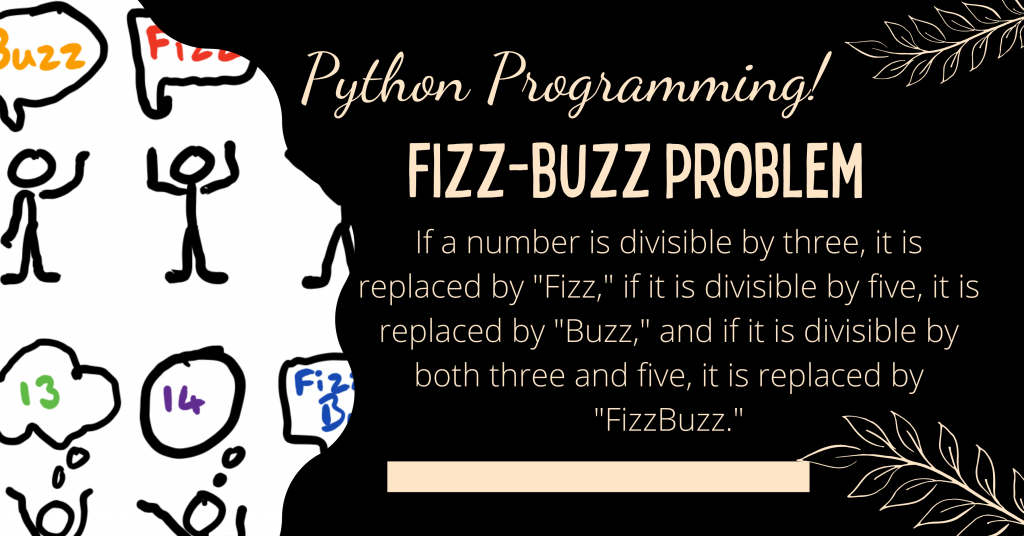# FizzBuzz Problem – Implementing the FizzBuzz algorithm in PythonThe FizzBuzz algorithm is a popular question in coding interviews. Fizz and Buzz are numbers that are multiples of 3 and 5.

In this tutorial, I’ll show you how to use the Python programming language to create the FizzBuzz algorithm.

## The FizzBuzz Algorithm

The FizzBuzz algorithm was inspired by a children’s game. For a long time, this method has been one of the most popular coding interview problems.

In this problem, you are given a range of numbers and must create output using the following rules:

1. If the number (x) is divisible by three, the result must be “Fizz.”
2. If the number (x) is divisible by five, the result must be “Buzz.”
3. If the number (x) is divisible by both three and five, the result must be “FizzBuzz.”

This coding problem is common with numbers 3 and 5, however, you may encounter more complicated numbers, but the reasoning for solving the problem remains the same.

## FizzBuzz Algorithm using Python

In order to implement the FizzBuzz problem, we will be following the steps mentioned below:

1. Now we are considering only positive integers so we will be using a while loop till the point the user enters a positive integer.
2. Now we will using a for loop from 1 to n.
• Everytime we encounter a multiple of 3 and 5, we will print ‘FizzBuzz’
• For multiples of 3, we print ‘Fizz’
• And similary, for multiples of 5, we display the word ‘Buzz’
```n = -1
while(n<0):
n = int(input("Enter the ending integer: "))

for i in range(1, n+1):
if i % 3 == 0 and i % 5 == 0:
print("FizzBuzz",end=" ")
elif i % 3 == 0:
print("Fizz", end= " ")
elif i % 5 == 0:
print("Buzz", end = " ")
else:
print(i, end = " ")
```

## Sample Outputs

```Enter the ending integer: 20
1 2 Fizz 4 Buzz Fizz 7 8 Fizz Buzz 11 Fizz 13 14 FizzBuzz 16 17 Fizz 19 Buzz
```
```Enter the ending integer: 100
1 2 Fizz 4 Buzz Fizz 7 8 Fizz Buzz 11 Fizz 13 14 FizzBuzz 16 17 Fizz 19 Buzz Fizz 22 23 Fizz Buzz 26 Fizz 28 29 FizzBuzz 31 32 Fizz 34 Buzz Fizz 37 38 Fizz Buzz 41 Fizz 43 44 FizzBuzz 46 47 Fizz 49 Buzz Fizz 52 53 Fizz Buzz 56 Fizz 58 59 FizzBuzz 61 62 Fizz 64 Buzz Fizz 67 68 Fizz Buzz 71 Fizz 73 74 FizzBuzz 76 77 Fizz 79 Buzz Fizz 82 83 Fizz Buzz 86 Fizz 88 89 FizzBuzz 91 92 Fizz 94 Buzz Fizz 97 98 Fizz Buzz
```

## Conclusion

Numbers that are divisible by 3 and 5 are referred to as fizz and buzz. If a number is divisible by three, it is replaced by “Fizz,” if it is divisible by five, it is replaced by “Buzz,” and if it is divisible by both three and five, it is replaced by “FizzBuzz.”

I hope you enjoyed this tutorial on the Python programming language’s implementation of the FizzBuzz algorithm.

Happy Learning! 😇
More tutorials are included below: